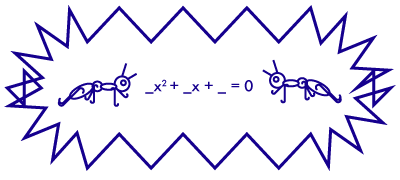Brilli the Ant is playing a game with Brian Till, her best friend. They are very competitive and always want to beat each other. Today, they are going to play the quadratic game.

Brilli is going to pick 3 non-zero real numbers and Brian is going to arrange the three numbers as the coefficients of a quadratic equation:

$\text{\_\_\_\_ }x^2 +\text{\_\_\_\_ }x +\text{\_\_\_\_} = 0.$

Brilli wins the game if and only if the resulting equation has two distinct rational solutions.

Who has a winning strategy?

×